## Figures index

#### Shyam Sundar

American Journal of Applied Mathematics and Statistics. 2015, 3(2), 59-67 doi:10.12691/ajams-3-2-3• Figure 1. Nonlinear stability in B-N plane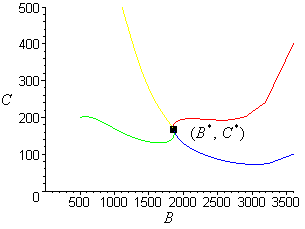• Figure 2. Nonlinear stability in B-C plane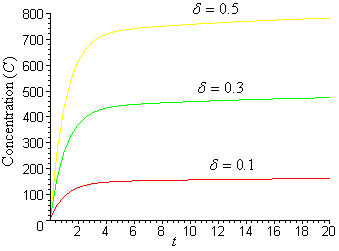• Figure 3. Variation of concentration of CO2 with time 't' for different values of δ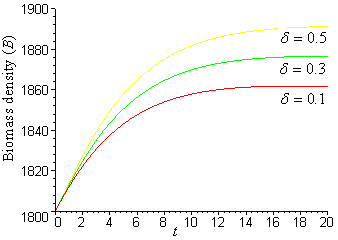• Figure 4. Variation of biomass density with time 't' for different values of δ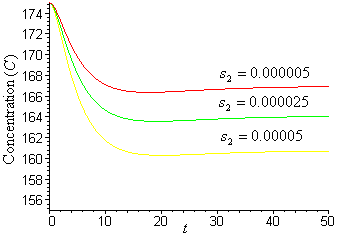• Figure 5. Variation of concentration of CO2with time 't' for different values of S2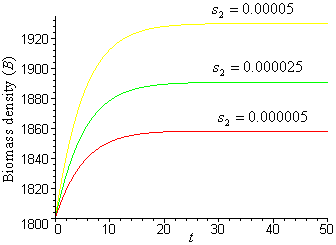• Figure 6. Variation of biomass density with time 't' for different values of S2• Figure 7. Variation of biomass with time 't' for different values of r1• Figure 8. Variation of population density with time 't' for different values of r1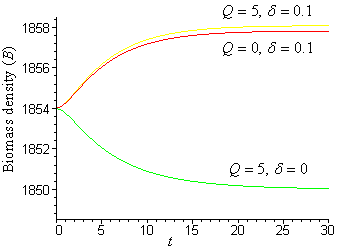• Figure 9. Variation of biomass (B)with time 't' for different values of Q and δ• Figure 10. Variation of concentration of CO2 with time 't' for different values of Q and δ